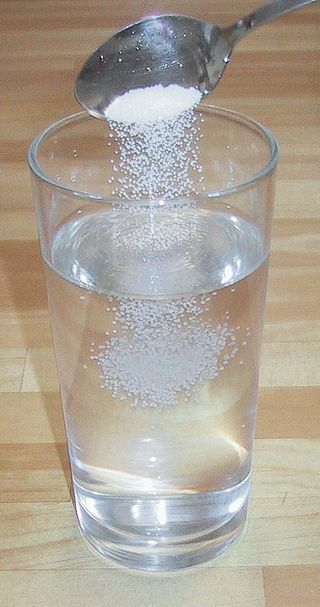# 13.2: Solutions: Homogeneous Mixtures

skills to develop

• Learn some terminology involving solutions.
• Explain the significance of the statement "like dissolves like".
• Explain why certain substances dissolve in other substances.

The major component of a solution is called the solvent. The minor component of a solution is called the solute. By major and minor we mean whichever component has the greater presence by mass or by moles. Sometimes this becomes confusing, especially with substances with very different molar masses. However, here we will confine the discussion to solutions for which the major component and the minor component are obvious.

Solutions exist for every possible phase of the solute and the solvent. Salt water, for example, is a solution of solid $$\ce{NaCl}$$ in liquid water, while air is a solution of a gaseous solute (O2) in a gaseous solvent (N2). In all cases, however, the overall phase of the solution is the same phase as the solvent. Table $$\PageIndex{1}$$ lists some common types of solutions, with examples of each.

Table $$\PageIndex{1}$$: Types of Solutions
Solvent Phase Solute Phase Example
gas gas air
liquid gas carbonated beverages
liquid liquid ethanol (C2H5OH) in H2O (alcoholic beverages)
liquid solid salt water
solid gas H2 gas absorbed by Pd metal
solid liquid Hg(ℓ) in dental fillings
solid solid steel alloysFigure $$\PageIndex{1}$$: Making a saline water solution by dissolving table salt (NaCl) in water. The salt is the solute and the water the solvent. Image used with permission (CC-BY-SA 3.0; Chris 73).

Example $$\PageIndex{1}$$: Sugar and Water

A solution is made by dissolving 1.00 g of sucrose ($$\ce{C12H22O11}$$) in 100.0 g of liquid water. Identify the solvent and solute in the resulting solution.

Solution

Either by mass or by moles, the obvious minor component is sucrose, so it is the solute. Water—the majority component—is the solvent. The fact that the resulting solution is the same phase as water also suggests that water is the solvent.

Exercise $$\PageIndex{1}$$

A solution is made by dissolving 3.33 g of $$\ce{HCl(g)}$$ in 40.0 g of liquid methyl alcohol ($$\ce{CH3OH}$$). Identify the solvent and solute in the resulting solution.

solute: HCl(g); solvent: CH3OH

## Like Dissolves Like

A simple way to predict which compounds will dissolve in other compounds is the phrase "like dissolves like". What this means is that polar compounds dissolve polar compounds, nonpolar compounds dissolve nonpolar compounds, but polar and nonpolar do not dissolve in each other.

Even some nonpolar substances dissolve in water but only to a limited degree. Have you ever wondered why fish are able to breathe? Oxygen gas, an nonpolar molecules, does dissolve in water and it is this oxygen that the fish take in through their gills. Or, one more example of a nonpolar compound that dissolves in water is the reason we can enjoy carbonated sodas. Pepsi-cola and all the other sodas have carbon dioxide gas, $$\ce{CO_2}$$, a nonpolar compound, dissolved in a sugar-water solution. In this case, to keep as much gas in solution as possible, the sodas are kept under pressure.

This general trend of "like dissolves like" is summarized in the following table:

Table $$\PageIndex{1}$$: Summary of Solubilities
Solute Solvent Is Solution Formed?
Polar Covalent Polar yes
Non-polar Covalent Non-polar yes
Polar Covalent Non-polar no
Non-polar Covalent Polar no
Ionic Polar yes
Ionic Non-polar no

Note that every time charged particles (ionic compounds or polar substances) are mixed, a solution is formed. When particles with no charges (nonpolar compounds) are mixed, they will form a solution. However, if substances with charges are mixed with other substances without charges a solution does not form.

When an ionic compound is considered "insoluble", it doesn't necessarily mean the compound is completely untouched by water. All ionic compounds dissolve to some extent. An insoluble compound just doesn't dissolve in any noticeable or appreciable amount.

What is it that makes a solute soluble in some solvents but not others?

The answer is intermolecular interactions. The intermolecular interactions include London dispersion forces, dipole-dipole interactions, and hydrogen bonding (as described in Chapter 10). From experimental studies, it has been determined that if molecules of a solute experience the same intermolecular forces that the solvent does, the solute will likely dissolve in that solvent. So, NaCl—a very polar substance because it is composed of ions—dissolves in water, which is very polar, but not in oil, which is generally nonpolar. Nonpolar wax dissolves in nonpolar hexane, but not in polar water.Figure $$\PageIndex{2}$$: Water (clear liquid) and oil (yellow) do not form liquid solutions. Image used with permission (CC BY-SA 1.0 Generic; Victor Blacus)

Example $$\PageIndex{2}$$: Polar and Nonpolar Solvents

Would I2 be more soluble in CCl4 or H2O? Explain your answer.

Solution

I2 is nonpolar. Of the two solvents, CCl4 is nonpolar and H2O is polar, so I2 would be expected to be more soluble in CCl4.

Exercise $$\PageIndex{2}$$

Would C3H7OH be more soluble in CCl4 or H2O? Explain your answer.

H2O because both experience hydrogen bonding

Example $$\PageIndex{3}$$

Water is considered a polar solvent. Which substances should dissolve in water?

1. methanol (CH3OH)
2. sodium sulfate (Na2SO4)
3. octane (C8H18)

SOLUTION

Because water is polar, substances that are polar or ionic will dissolve in it.

1. Because of the OH group in methanol, we expect its molecules to be polar. Thus, we expect it to be soluble in water. As both water and methanol are liquids, the word miscible can be used in place of soluble.
2. Sodium sulfate is an ionic compound, so we expect it to be soluble in water.
3. Like other hydrocarbons, octane is nonpolar, so we expect that it would not be soluble in water.

Exercise $$\PageIndex{3}$$

Toluene (C6H5CH3) is widely used in industry as a nonpolar solvent. Which substances should dissolve in toluene?

1. water (H2O)
2. sodium sulfate (Na2SO4)
3. octane (C8H18)